## Register Now

Lorem ipsum dolor sit amet, consectetur adipiscing elit.Morbi adipiscing gravdio, sit amet suscipit risus ultrices eu.Fusce viverra neque at purus laoreet consequa.Vivamus vulputate posuere nisl quis consequat.

Grading of Underground Cables is essential for uniform Electrostatic Stress distribution in the cable. Unreliable or inconsistent Electrostatic Stress distribution in a cable is undesirable as it requires insulation of greater thickness which increases the cable size. This post will discuss Grading of Underground Cables, its types i.e. Capacitance and Inter-Sheath Grading, advantages, disadvantages and Dielectric Stress.

## What is Grading of Underground Cables

Grading is defined as the method of equalizing the Electrostatic Stress in the dielectric of the cable. Grading makes the cable of the same size to function at operational voltage and also at higher voltages.

Grading of Underground Cable is necessary for uniform Electrostatic Stress distribution in the cable.  If the stress distribution is uneven, then there is a high chance of breakdown in outer insulation layer. One may think that if the thickness of the insulation layer is increased, then it may prevent breakdown. Yes, but doing so will increase the cable size which is undesirable. The mot suitable way to keep a uniform stress distribution in underground cable is Grading.Fig. 1 – Underground Cables

This is achieved using two methods of Grading namely:

To understand the concept of Grading of Underground Cables, knowledge of Dielectric Stress becomes a pre-requisite.

## What is Dielectric Stress in a Single-Core Cable

The maximum Electric Field or the Electrostatic Stress that an insulating material can withstand without losing its insulating properties is described as the Dielectric Stress. It may also be defined as the maximum voltage required to produce a dielectric breakdown through the material.

It is expressed in terms of Volts per Unit Thickness. It is also a measure of Potential Gradient at a specific point.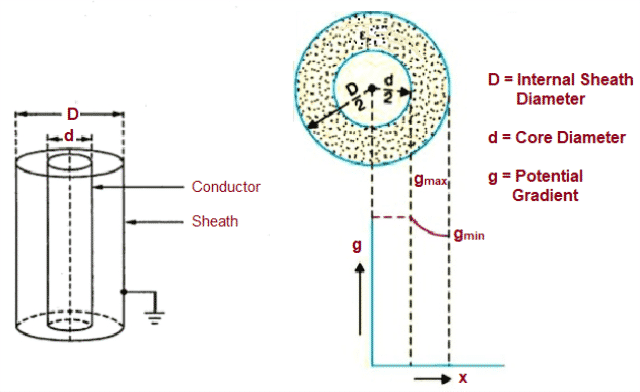Fig. 2 – Dielectric Stress in a Cable

From the above figure, Potential Gradient (g) at a point (x) meters from the center of the cable can be calculated using the equation,Where, Ex = Electric Intensity and by definition g = Ex

The potential difference (V) between conductor and sheath is calculated using the equation,By substituting the value of Q, Potential Gradient (g) is obtained as shown in the equation below. It also states that Potential Gradient (g) is inversely proportional to the Distance (x).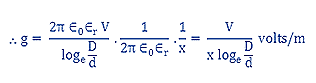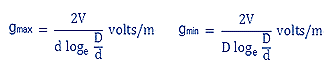The ratio of gmax and gmin  is:The surface of the conductor experiences maximum variation of stress and reduces considerably for the layers away from the conductor. It is an important factor when designing a cable.

## Types of Grading of Underground Cable

Grading of Underground Cables may be categorized in two types, i.e. :

Capacitance Grading is achieved by using composite dielectric i.e. various layers of different dielectric are arranged to ensure that the Potential Gradient remains constant. It is inversely proportional to its distance from the centre.

Using this, a uniform dielectric stress is maintained in the cable. Generally two or three dielectrics having different Relative Permittivity is used. The dielectric with highest permittivity is placed near the core of the cable and subsequently the other dielectric layers are arranged in the decreasing order of permittivity.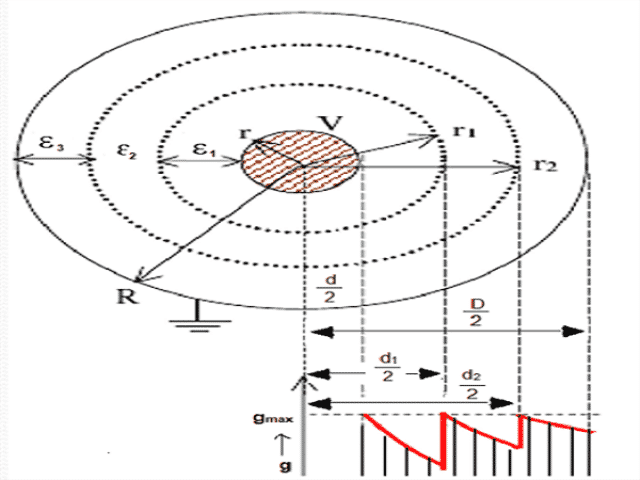In the above figure, the three dielectric layers have been consideres whose inner radii is r, r1, r2 and outer diameters is d, d1, d2. The Relative Permittivity of the layers are ε1, ε2, ε3 respectively. If the three dielectric layers are subjected to maximum stress then the potential difference of the layers are: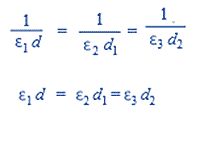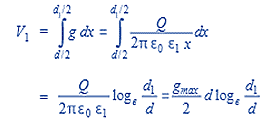Similarly, the potential difference across the other two layers V2, V3 are: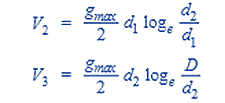The total Potential Difference between Core and the Outer Sheath is V = V1 + V2 + V3We can conclude that by grading the insulation, cable works at a greater potential compared to non- graded cable without increasing the diameter of the cable.

Inter-Sheath Grading makes use of Homogeneous Dielectric. It is divided into different layers by inserting metallic Inter-Sheaths between the Core and Outer Sheath. This provides a channel for charging current. The metallic Inter-Sheaths are maintained at adequate voltage levels as they are connected to the Auxiliary Transformer. This ensures uniform Potential Gradient throughout the dielectric of the cable.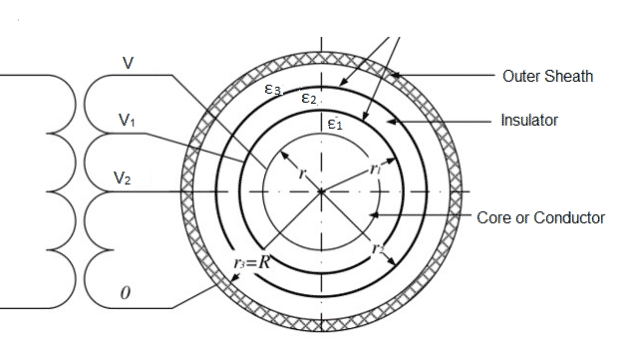In the above figure, the three layers of Insulation are separated by two Inter-Sheaths at definite voltages ‘V1, ‘V2’ and the Core or Conductor voltage is at ‘V’. As each Inter-Sheath layer experiences different Potential levels it can be treated as a homogeneous single core cable.

Hence the stress is calculated for each layer using the equation :As the Dielectric used is homogeneous the stress in each layer is equal. Hence, g1 max = g2 max  and the voltage between the Conductor and Outer Sheath is V = V1 + V2

We can conclude that all the potentials are in phase as the cable functions like three capacitors in series. Also, the presence of charging currents results in considerable losses in Inter-Sheath layers.

• Due to reduced dielectric thickness, the cable size is smaller.
• Chances of dielectric failure is reduced in Capacitance Grading.
• Dielectric loss is less at lower voltages.

```Also Read: